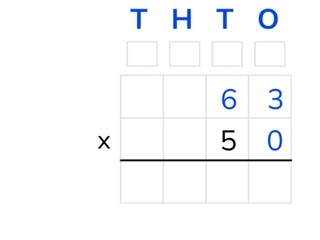Standard algorithm multiplication with tens and numbers to 100

# Standard algorithm multiplication with tens and numbers to 100

Students learn standard algorithm multiplication with tens and numbers to 100.

No account needed.8,000 schools use Gynzy92,000 teachers use Gynzy1,600,000 students use Gynzy

## General

Students learn standard algorithm multiplication with a tens number and a number to 100.

## Standards

CCSS.Math.Content.5.NBT.B.5

## Learning objective

Students will be able to multiply using the standard algorithm, with a tens number and a number to 100.

## Introduction

The students solve two problems, for which they must multiply with a tens number and a number to 100.

## Instruction

Explain that with standard algorithm multiplication you start by putting the numbers one under the other. You put the larger number on top. The T, H, T, O above the chart refer to the thousands, hundreds, tens, and ones. Show that above each column there is a regrouping box. In these boxes you can write down regrouped numbers and add them up with the other outcomes later. Explain that with standard algorithm multiplication with a tens number you can write down a 0 in the ones place, because all multiplications with this number make 0. Briefly show that this is true for the problems 0 x 3 and 0 x 6. Now multiply the lower number from the tens (T) column and the higher number from the ones (O) column. Write down the 5 under the line in the tens (T) column and the 1 in the regrouping box from the hundreds (H). Next you multiply the lower number from the tens (T) column with the higher number from the tens (T) column (5 x 6 = 30). Emphasize that you add the 1 from the regrouping box to your answer (30 + 31 = 31). Write this answer under the line in the hundreds and thousands columns. Practice two problems together with the students that must be solved using the standard algorithm. Students may use a sheet of scrap paper. The students solve the next three problems on their own.

Check whether the students can multiply using the standard algorithm with a tens number and a number to 100, by asking the following question:
- What steps do you take to solve the problem 80 × 54 using the standard algorithm?

## Quiz

The students test their understanding of standard algorithm multiplication with a tens number and numbers to 100 through ten exercises. For some the THTO chart has been filled in with the numbers of the problem, and for others the students must set up the problem on their own.

## Closing

Discuss once again the importance of being able to multiply using the standard algorithm with a tens number and a number to 100. As a closing activity you can have the students solve problems working in pairs, for which they can write their answers on the board. Then the answers can be checked together.

## Teaching tips

Have students that have difficulty with standard algorithm multiplication with a tens number and a number to 100, first practice multiplying with simple numbers and a tens number. Next you use a similar type of problem in the chart to solve it using the standard algorithm.

## Instruction materials

Scrap paper

### The online teaching platform for interactive whiteboards and displays in schools

• Save time building lessons

• Manage the classroom more efficiently

• Increase student engagement